Next: Implementation Up: Laguerre method Previous: Laguerre method   Contents

Mathematical background

Let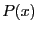be an univariate polynomial of degree: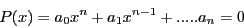with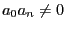,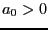. Let's define the sequence :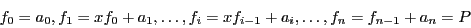If it exists a real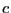such that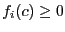for all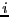in [0,], then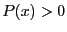for all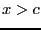. Consequently all the roots of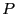are lower than. To findthe following scheme can be used:
1. letbe such that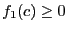2. let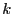the smallest integer such that either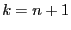or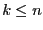and3. ifthen substituteby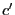such that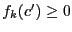and go to 2
4. returnA consequence of Laguerre theorem is that the best bound cannot be lower than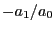.

Jean-Pierre Merlet 2012-12-20